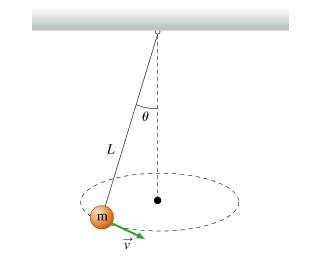# Finding tangential speed to maintain an angle theta

1.What tangential speed, v, must the bob have so that it moves in a horizontal circle with the string always making an angle theta from the vertical?
Express your answer in terms of some or all of the variables m, L, and theta, as well as the acceleration due to gravity g.

2. I figured out the following:

T = mg/cos(theta)
r = Lsin(theta)
a_c = v^2/Lsin(theta)

3. see above

I next had to get the horizontal (inward radial) force, which is mgtan(theta). How was that force obtained? Also, how do I use this information to answer the question?

## Answers and Replies

Second part of question: How long does it take the bob to make one full revolution (one complete trip around the circle)?

I know that the speed is constant = sqrt[Lgsin(theta)tan(theta), and I also know the distance is the circumference of the circle = 2*pi*Lsin(theta). I know I have to use kinematics but which equation?